# To sort, or not to sort ( Bubble, Quick )

Out there, there are tons of algorithms which are intended to fulfill one main goal, to sort. Nobody knows the exact number of how much algorithms of this kind exist and each of them overreaches the target in a different, more or less timesaving, way. What they still have in common is, that they take some bunch of data and bring it in a proper order.
As there exist so many sorting algorithms, this article is concerned with the analysis of two rather popular recursive ones. Especially aspects related to the performance and the operation principles of each algorithm will be examined.
Before you start reading I would strongly recommend you to get yourself a short introduction into Landau-notation or just the idea behind the use of Big O,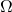andnotation, as we will make use of the basics here.

First of all I will introduce a subroutine used by each of the sorting algorithms, as it is used to swap the position of two elements within an Array or some similar constructed data structure.

SWAP(A : [1...n], i1, i2)

# Costs Count
1. tmp = A[i1]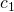2. A[i1] = A[i2]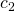3. A[i2] = tmpSumming up the costs of each line makes it possible to calculate the runtime for SWAP.

(1)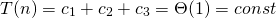As the costs for each step of the routine are constant and each step is executed exactly 1 time, the total runtime of SWAP is asymptotically constant. I will refer to this section later on, when SWAP is used.

## Bubble Sort

The first algorithm is one very simple and therefore kinda slow one, which just goes through a set of data and compares each member with its right neighbour. Dependent on their values, the higher valued participant moves right and swaps position with its less valued neighbour. If the order of the dataset changes during a run of Bubblesort the sorting will be repeated until the last run recognizes no more changes.

Knowing the principle of the algorithm makes it possible to describe it in a more analytical way, using Pseudo-Code. The following table displays the costs the execution of each line of Pseudo-Code requires and the times it will be executed on each call of the routine.

BUBBLESORT(A : [1...n], iteration : increments on each call)

# Costs Count
BC WC
1. changed = false2. for i = 1 to A.length – iteration inclusive3.   if A[i] > A[i+1]4.     SWAP(A, i, i+1)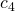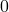5.     changed = true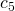6. if changed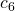7.   BUBBLESORT(A, iteration+1)Our next step is to predict the runtime of the routine. But first we have to face one remaining problem. Because of the conditional recursiveness of the algorithm, it is not possible to be 100% sure if the algorithm calls itself again. The solution for this problem is to observe the best and the worst case runtime separately, as done in the separate columns.

The best case occurs when the array is already sorted. In this case line 4., 5. and 7. will never be executed and it is possible to calculate the runtime easily, because the total runtime equals the runtime of one run.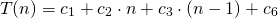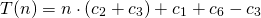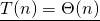While the runtime of the best case was easy to calculate, it turns out that, the worst case scenario is far more complicated to analyse. This scenario appears when the array is in reverse order.
Taking a look at the first 6 lines allows us to predict the non-recursive part of the runtime in the same way as used for the best case runtime.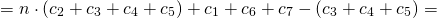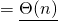But as the routine calls itself again and again in line 7, we have to solve the following recursion equation in order to define a total runtime for the worst case scenario.

(2)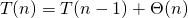While the first part was just the sum of all costs times their occurences, the solution for the recursive part of the equation is a little bit trickier to find.
We have to imagine a recursion tree oflevels, with a runtime of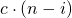on the ith level, for all. So we get the total runtime by summing up every levels runtime, multiplying the result with the height of the tree.

(3)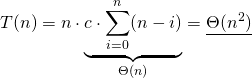Putting the results for both extreme cases together, makes it possible to narrow the runtime of BUBBLESORT for input in any order.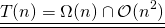## Quick Sort

Bubblesort is a nice example to get into sorting algorithms, but actually it is a little bit boring. So you may be glad to read that, we now attend to one more sophisticated exemplar, the Quicksort.
Quicksort basically runs through 4 steps.

1. Pick a pivot element out of the input.
2. Find one element less than the pivot element iterating from the left and one with a higher value starting from the right.
3. Swap the two elements.
4. Split the input in two parts and do the whole thing again using them as the input, using the pivot elements correct position as the delimiter.

As you can see, the principle of Quicksort is not very difficult to get. The only opportunity to make it a bit more fascinating, is the way you choose the pivot element. It turns out that, statistically it does not matter which element you take, but you have to keep in mind which one you chose, because it could affect the implementation.
I will use the element in the center of the input as the pivot element, also we have to know that Quicksort sorts in-place, i.e. there will be just the original input and no copy of it, thus the input will not have to be merged again after the sorting is done. As the sorting will be done in-place we need to delimit the part of the input the routine is currently working on in another way. We do this by requiring additional input, the two delimiting indices first and last. This time we can not use the size of the input to calculate the count each line is executed, so we have to definie one auxiliary variable to obtain the size of the currently processed part of the input.Now we have enough information to define the whole thing in pseudo code and to predict a significant runtime for it.

QUICKSORT(A : [1...m], first, last)

# Costs Count
BC WC
1. if first < last2.   pivot = A[(last + first) / 2]3.   i = first4.   j = last5.   while i ≤ j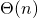6.     while A[i] < pivot7.       i = i + 1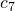8.     while A[j] > pivot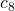9.       j = j – 1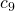10.     if i ≤ j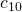11.       SWAP(A, i+ +, j- -)12.   QUICKSORT[A, first, i - 1]13.   QUICKSORT[A, i, last]Separating the analysis into cases makes it again possible to limit the possible runtime for any input. As both extreme cases occur for various inputs and the lines 5 to 11 are executed at a max of a multiple of linear, it suffices to say that, those lines aretimes executed. The interesting part happens in the lines 12 and 13 where the input is separated. Thus the runtime majorly depends on the size of each separated part.
At first we observe the best case, which appears when the pivot element is already on its correct position and the input is splitted into two parts of equal size. Because Quicksort is recursive, first of all we have to calculate the non-recursive required amount of runtime, which is needed to sort the currently processed part of the input.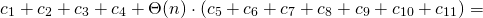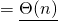This gives us the recursion equation for the best case.

(4)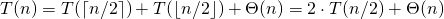As the equation matches the pattern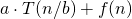it is possible to apply the Mastermethod to solve the equation. Drawing the recursion tree would be a similarly easy way to resolve the equation.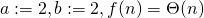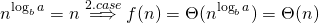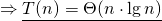While the best case occurs when the input is splitted into two parts of most balanced size, the worst case occurs when the size of each part is maximum unbalanced. In other words, when the size of the first part equalsand the second part is empty.
As the non-recursive amount of runtime equals the one calculated for the best case, we get the following recursion equation for Quicksort in worst case.

(5)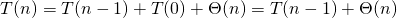Now we have the same situation as in the worst case scenario of Bubble Sort. So we just have to use equation (3) to predict a runtime for the worst case scenario of Quicksort.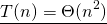Summarizing our new insights, we are able to predict Quicksorts runtime in case of any input.If you liked this article on the mathematical analysis of Quicksort and Bubblesort, I request you to support it by voting it up at Hacker News.